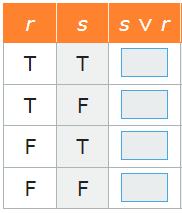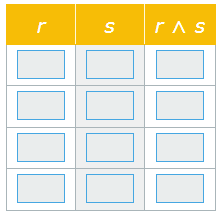Name the Postulates/Theorems/Properties
Truth Tables
Counterexamples
100

What is the converse of this statement? Original: If the beach ball pops, Then it is flat.

If it is flat, Then the beach ball popped.

100

Choose the property that justifies the following.

-3x+5+2x=12 and x=4

-3(4)+5+2(4)=12

What is...

Substitution Property.

100

If p is a true statement and q is a false statement, what is the truth value of

p implies q

False

100

Provide a counterexample:

If you are eating a red fruit, then you are eating an apple.

pomegranate, strawberry, raspberry, cherry, etc

200

If the sun is NOT shining, Then we are inside watching tv. What is the Inverse of this statement?

If the sun is shining, Then we are not inside watching tv.

200

Segment AB is congruent to Segment BA. This is an example of...

Symmetric Property of Equality.

200

If p is a false statement and q is a true statement, what is the truth value of

p wedge q

False

200

Provide a counterexample

If 𝑥 + 4 > 10, then x is greater than 8.

6.1, 6.5, 7, 7.3, 7.555555, 7.9999

300

If we are in Biology, Then we are in school. What is the contrapositive?

If we are not in school, then we are not in biology.

300

Decide is the following statement is true always, sometimes, or never.

A line contains 3 or more points.

What is...

sometimes.

300

If p is a true statement and q is a false statement, what is the truth value of

~(p vee q)

False

300

Provide a counterexample:

If angle ABC is obtuse, then it is equal to 100°.

False

Counterexample: an angle equal to 145°

400

What phrase does a biconditional contain?

the phrase "if and only if"

400

Which property is illustrated by the following statement: If (a+b) = (d+e), then (a+b) - c = (d+e) - c

Subtraction Property of Equality

400

Fill in the following blanks for the truth table below.T, T, T,f

400

Provide a counterexample:

If x²=16, then x=4.

False, x = -4

500

What does negation mean and what is its symbol?

Symbol: ~

Means "not"

500

What does the Segment Addition Postulate state?

What is...

If B is between A and C, then AB + BC = AC.

OR

If AB + BC = AC, then B is between A and C.

500

Fill in the following blanks for the truth table below.T, F, F, F

500

Provide a counterexample to the following statement. "All lines will eventually intersect with each other."

False. Parallel lines will never intersect.

Click to zoom